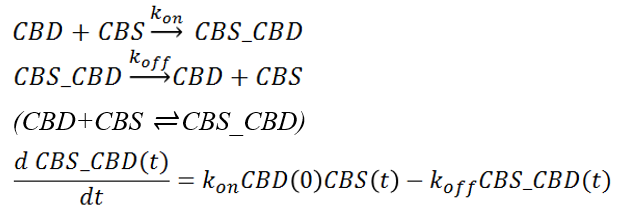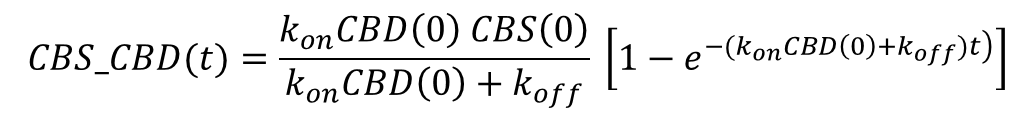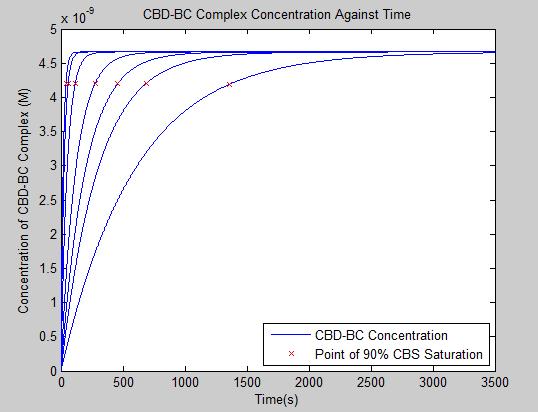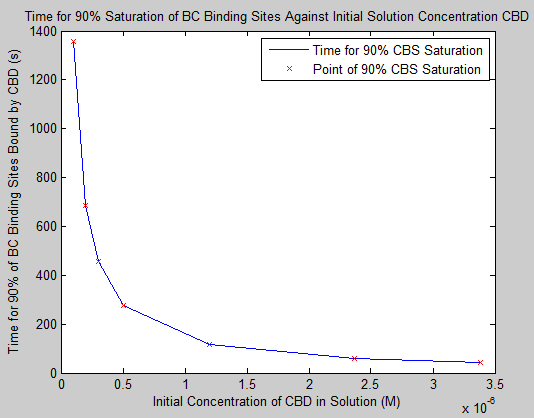# Team:Imperial/CBD Kinetics Model

Imperial iGEM 2014

# CBD Kinetics Model

## Introduction

Based on a set of ordinary differential equations (ODEs) describing the kinetics of carbohydrate-binding domains (CBDs) attaching to bacterial cellulose (BC), the model is designed to predict the efficiency and affinity of the binding process. Given an initial concentration of CBD injected into the system, the model calculates the time necessary for a certain percentage of protein binding sites on BC to be saturated.

With a better understanding of the interaction between CBD and BC, wetlab experiments can be informed about the functionalisation capability of BC and therefore would be able to approximate efficiency of the contaminants-capturing process.

## Mathematical Background

• Dependent Variables:
• CBS(t): concentration of unoccupied cellulose binding sites (CBSs) at time t
• CBD(t): concentration of unbound CBD at time t
• CBS_CBD(t): concentration of binding complex (CBS bounded with CBD) at time t
• Parameters:
• CBS(0): initial concentration of CBS
• CBD(0): initial concentration of CBD injected into the system
• kon: association rate constant
• koff: dissociation rate constant
• KD = koff/kon: equilibrium dissociation constant
• Binding Kinetics:
•• Assume that CBD(t) = CBD(0) since the initial concentration of CBD injected into the system is typically very large compared to the initial concentration of cellulose binding sites, i.e. CBS(0).
• Initial and Boundary Conditions:
• CBD(0) = M0, where M0 is a value determined as per experiment.
• CBS_CBD(0) = 0
• CBS_CBD(∞) = CBS(0), assume that BC binding sites can be fully saturated given enough time, as konCBD(0) >> koff.
• Solution:
•• where kon CBD(0) >> koff

## Interaction with wetlab experiments

Parameters koff and kd are determined by wetlab assays (wash experiments), from which kon is calculated: kon=koff/kd.

With the parameters determined from the experiments, the model is then instantiated and used to predict the time period necessary for a certain percentage of the binding sites to be saturated. The validity of the model is a posteriori checked through further wetlab experiments (please refer to CBD expression experiments for further information).

The chart below illustrates how our model and the wetlab experiments interact with each other.

## Results and ConclusionsFigure 1: CBD-BC complex concentration against timeFigure 2: Time for 90% saturation of BC binding sites against initial concentration of CBD

It can be observed from the second graph that the time necessary for 90% CBS saturation decreases significantly when initial CBD concentration increases beyond 0.5 µM; however, a further increase in initial CBD concentration does not contribute much to effectively reduce the time necessary for 90% CBS saturation. Therefore, an initial CBD concentration between 0.5 µM and 1 µM will be considered as optimal to efficiently functionalise BC.

## Appendix: Code

The code for this model can be found here: CBD_kinetics_model_imperial_iGEM_2014.m